In Progress
Lesson 11 of 24
In Progress

# The Limit Laws

In the previous section, we evaluated limits by looking at graphs or by constructing a table of values. In this section, we establish laws for calculating limits and learn how to apply these laws. In the Student Project at the end of this section, you have the opportunity to apply these limit laws to derive the formula for the area of a circle by adapting a method devised by the Greek mathematician Archimedes. We begin by restating two useful limit results from the previous section. These two results, together with the limit laws, serve as a foundation for calculating many limits.

# Evaluating Limits with the Limit Laws

The first two limit laws were stated in (Figure) and we repeat them here. These basic results, together with the other limit laws, allow us to evaluate limits of many algebraic functions.

We now take a look at the limit laws, the individual properties of limits. The proofs that these laws hold are omitted here.

# Limits of Polynomial and Rational Functions

By now you have probably noticed that, in each of the previous examples, it has been the case that. This is not always true, but it does hold for all polynomials for any choice ofand for all rational functions at all values offor which the rational function is defined.

To see that this theorem holds, consider the polynomial. By applying the sum, constant multiple, and power laws, we end up with

It now follows from the quotient law that ifandare polynomials for which, then.

# Additional Limit Evaluation Techniques

As we have seen, we may evaluate easily the limits of polynomials and limits of some (but not all) rational functions by direct substitution. However, as we saw in the introductory section on limits, it is certainly possible forto exist whenis undefined. The following observation allows us to evaluate many limits of this type:

If for allover some open interval containing, then.

To understand this idea better, consider the limit.

The function

and the functionare identical for all values ofThe graphs of these two functions are shown in (Figure).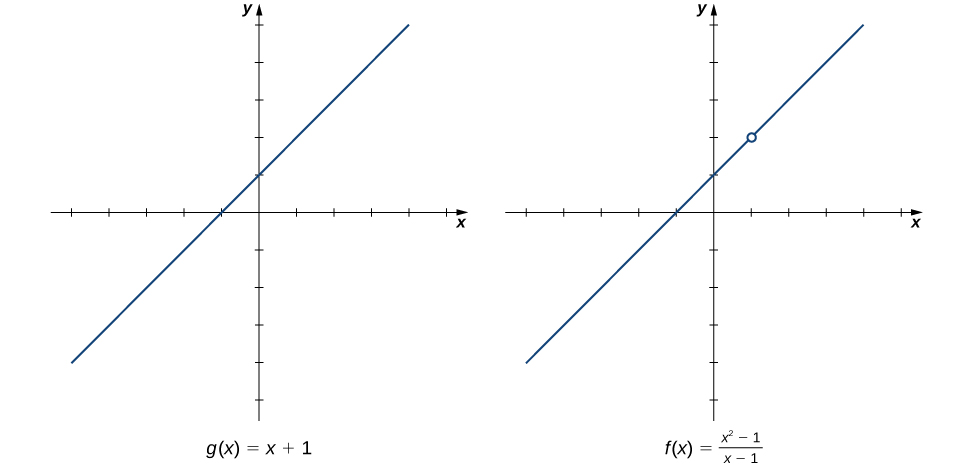Figure 1. The graphs of  and  are identical for all . Their limits at 1 are equal.

We see that

The limit has the form, whereand. (In this case, we say thathas the indeterminate form 0/0.) The following Problem-Solving Strategy provides a general outline for evaluating limits of this type.

In (Figure) we look at one-sided limits of a piecewise-defined function and use these limits to draw a conclusion about a two-sided limit of the same function.

### Evaluating a Two-Sided Limit Using the Limit Laws

Forevaluate each of the following limits:

1.2.3.#### Solution

(Figure) illustrates the functionand aids in our understanding of these limits.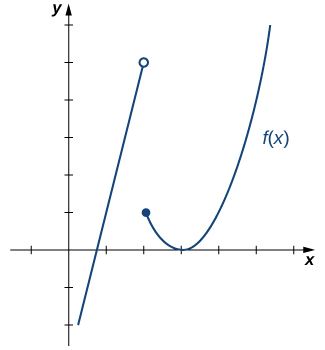=2, with the equation (x-3)^2. There is a closed circle at (2,1). The vertex of the parabola is at (3,0).” width=”325″ height=”350″> Figure 3. This graph shows the function .
1. Sincefor allin, replacein the limit withand apply the limit laws:.
2. Sincefor allin, replacein the limit withand apply the limit laws:.
3. Sinceand, we conclude thatdoes not exist.

# The Squeeze Theorem

The techniques we have developed thus far work very well for algebraic functions, but we are still unable to evaluate limits of very basic trigonometric functions. The next theorem, called the squeeze theorem, proves very useful for establishing basic trigonometric limits. This theorem allows us to calculate limits by “squeezing” a function, with a limit at a pointthat is unknown, between two functions having a common known limit at(Figure) illustrates this idea.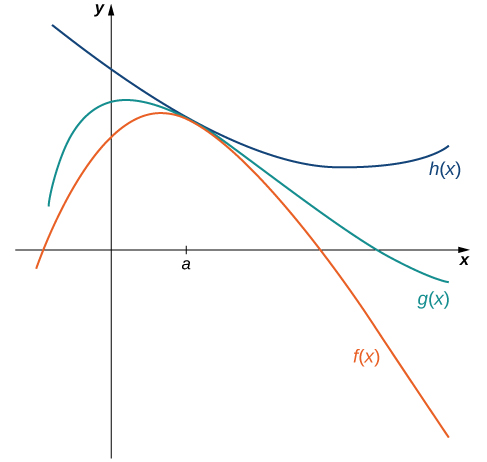Figure 4. The Squeeze Theorem applies when  and .

We now use the Squeeze Theorem to tackle several very important limits. Although this discussion is somewhat lengthy, these limits prove invaluable for the development of the material in both the next section and the next chapter. The first of these limits is. Consider the unit circle shown in (Figure). In the figure, we see thatis the-coordinate on the unit circle and it corresponds to the line segment shown in blue. The radian measure of angle θ is the length of the arc it subtends on the unit circle. Therefore, we see that for.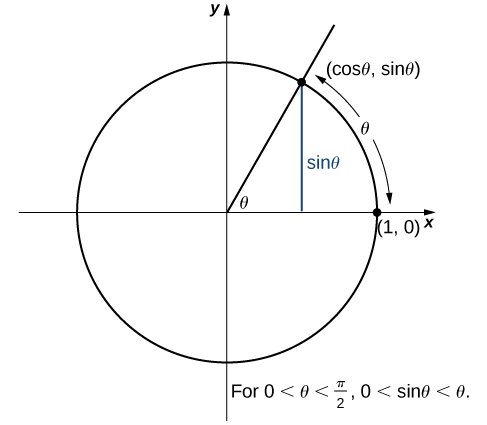Figure 6. The sine function is shown as a line on the unit circle.

Becauseand, by using the Squeeze Theorem we conclude that.

To see thatas well, observe that forand hence,. Consequently,It follows thatand,.

Next, using the identityfor, we see that.

We now take a look at a limit that plays an important role in later chapters—namely,. To evaluate this limit, we use the unit circle in (Figure). Notice that this figure adds one additional triangle to (Figure). We see that the length of the side opposite anglein this new triangle is. Thus, we see that for.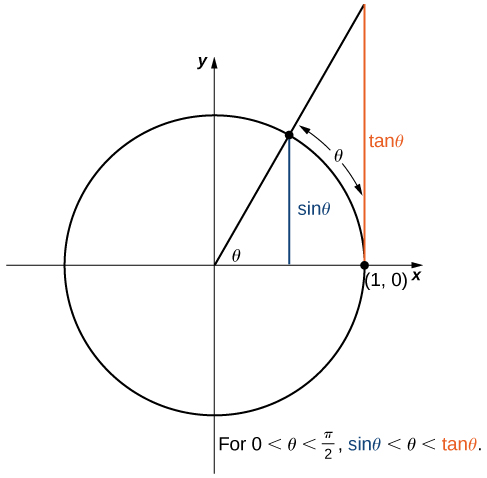Figure 7. The sine and tangent functions are shown as lines on the unit circle.

By dividing byin all parts of the inequality, we obtain.

Equivalently, we haveSince, we conclude that. By applying a manipulation similar to that used in demonstrating that, we can show that. Thus,.

In (Figure) we use this limit to establish. This limit also proves useful in later chapters.

# Key Equations

• Basic Limit Results• Important Limits